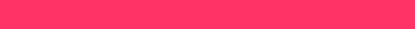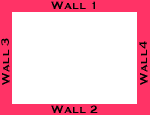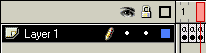# Flash Script – Ball and Wall Collision Effect

In this tutorial, I will show you how to create a Ball and wall collision effect.

I’ll use the simple `hittest()` function in Flash to achieve this effect. Download the sample files here. Let’s start working.

1. Open a new movie wiht a width=550 and a height=400.

2. First we will create the outer wall. Create a small rectangle object like this one:3. Convert the rectangle object to a movieclip, and name it "wall".

4. Now, drag four instances of the wall object and arrange as below.5. Give each wall an instance name. I used the instance names border1, border2, border3, and border4.

6. Now let’s create the ball. Create a small circle object.

7. Convert it to a movieclip, and give it an instance name. I called it "circle".

8. Now we will give the action script. Create three key frames as shown below.9. In the first key frame, instert the action:

``````   flag = 0;
//x coordinate =0
x= 190;
//y cordinate =0
y=190;
//random = 0
rand = 0;``````

10. Initialize the variable in the first key frame.

11. In the second key frame, insert these actions:

``````  //set the x and y property of the circle movieclip
setProperty ("/circle", _x, x);
setProperty ("/circle", _y, y);
// creates a random number with this function
function random_fun()
{
r = random(10);
return r;
}
// check if circle has hit border4 if hit change x and y values
if (circle.hittest(border4))
{
tellTarget ("/circle") {gotoAndPlay(2)}
flag = 1;
rand = random_fun()
}
// check if circle has hit border3 if hit change x and y values
if (circle.hittest(border3))
{
tellTarget ("/circle") {gotoAndPlay(2)}
flag = 2;
rand = random_fun()
}
// check if circle has hit border2 if hit change x and y values
if (circle.hittest(border2))
{
tellTarget ("/circle") {gotoAndPlay(2)}
flag = 3;
rand = random_fun()
}
// check if circle has hit border1 if hit change
x and y values
if (circle.hittest(border1))
{
tellTarget ("/circle") {gotoAndPlay(2)}
flag = 4;
rand = random_fun()
}
// set the x and y co-ordinates according the the
place where the circle hit
if (flag ==0) {
x = x+rand;
y = y+5;
}
if (flag == 1) {
x = x+5;
y = y-rand;
}
if (flag == 2) {
x = x-5;
y = y-rand;
}
if (flag == 3) {
x = x-rand;
y = y+5;
}
if (flag == 4) {
x = x + 5;
y = y - rand;
}
// end action script``````

12. In the third key frame insert the action:

``  gotoAndPlay (2);``

That’s it! The movie should now work!

This movie is not as complex as it looks. Actually, it’s very easy compared to others found on the Web. There are a lot of possibilities with this movie. Let your imagination run wild!